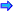# Algorithms

In the discussion above when STL was introduced, it was stated that STL is a library for containers, algorithms and iterators. Algorithms are functions that can act on containers or arrays and perform processes on the elements without having to write the functions to carry out the processes. In the brief STL introduction some of the algorithms were demonstrated. Below are listed only some of the algorithms in STL. There are in fact over 80 different algorithms and many are used infrequently.

The following table lists some of the more frequently used algorithms (in the table each begin and end is a pointer or iterator to the elements of the container object being acted upon and they represent the positions at which the function begins and ends its action on the elements of the container):Open table as spreadsheet

Algorithm

Purpose

binary_search(begin,end,value)

Returns the bool true if value is in the sorted sequence, and false otherwise.

find(begin,end,value)

Returns an iterator/pointer to position of value in the unsorted sequence or if not present returns the iterator end.

search(begin1,end1,begin2,end2)

Searches for the elements between begin2 and end2 in the range between begin1 and end1 and returns an iterator/pointer to the starting location if found and if the sequence of elements are not found end1 is returned.

count(begin,end,value)

Returns the number of times that value is found in the sequence.

copy(begin1,end1,begin2)

Copies the elements in the range from begin1 to end1 into the range starting at: begin2 (apparently the iterator begin2 must point to enough memory to permit copy of the data).

equal(begin1,end1,begin2,end2)

Returns the bool true if the two sequences are identical and false otherwise.

fill(begin,end,value)

Replaces all of the elements in the range with value.

merge(begin1,end1,begin2,end2,container1)

Merges the elements in the first sequence with the elements of the second sequence in container1.

replace(begin,end,old,new)

Replaces each occurrence of the element old with the element new.

reverse(begin,end)

Reverses the order of the elements in the range.

sort(begin,end)

Sorts the sequence of the elements in the container into ascending order.

unique(begin,end)

Removes all duplicates of an element in the container.Remember that the containers discussed above may be STL containers or arrays. Also remember that not all algorithms will work with all containers or arrays. Whether they work or not will depend on the particular container and on the underlying data type of the elements.

In the introduction to STL, examples of the sort( ), unique( ) and merge( ) algorithms were given. For additional examples of algorithms, check the following:

• For an example of the find( ) algorithm, see find.cpp.

• For an example of the count( ) algorithm, see count.cpp.

• For an example of the copy( ) algorithm, see copy.cpp

• For an example of the search( ) algorithm, see search.cpp.

One of the things you should notice when seeing a list of member functions for a particular container is that some of the member functions have the same name as some of the algorithm functions. To call a member function would require the use of the dot operator but calling an algorithm does not. A more important difference between these two types of functions is that the member function should be used rather than the algorithm because the member function has been fine tuned to work with that particular container.

As you look at the examples above as well as the examples from the introduction to STL, consider how much code had to be written using the built-in algorithms verses the code that would have had to be written if you did not have these algorithms written for you.